Question

# A box with a mass of 8.67 kg slides up a ramp inclined at an angle...

A box with a mass of 8.67 kg slides up a ramp inclined at an angle of 28.3° with the horizontal. The initial speed is 1.66 m/s and the coefficient of kinetic friction between the block and the ramp is 0.48. Determine the distance the block slides before coming to rest. m As shown in the figure below, a box of mass m = 35.0 kg is sliding along a horizontal frictionless surface at a speed vi = 5.55 m/s when it encounters a ramp inclined at an angle of θ = 28.4°. The coefficient of kinetic friction between the ramp and the box is μ = 0.0704 and the box slides a distance d up the ramp before coming momentarily to rest. Determine the distance the box slides up the ramp before coming momentarily to rest. m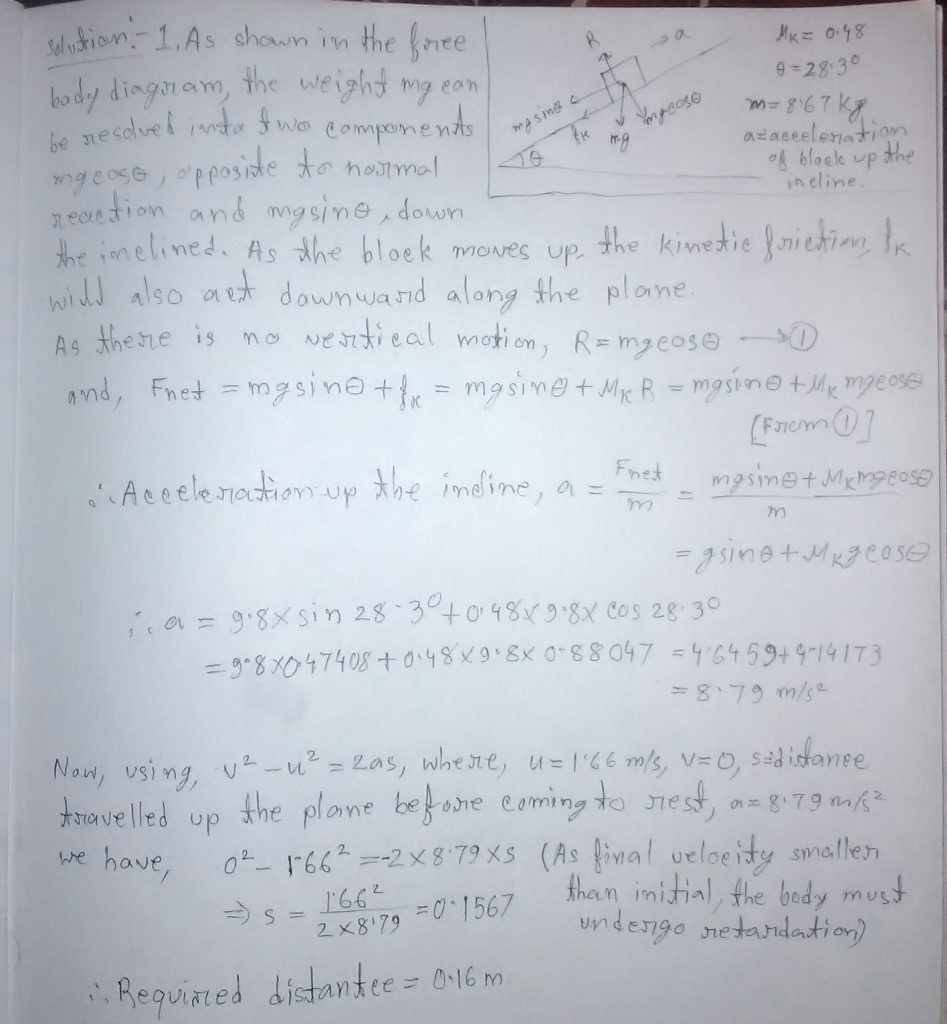#### Earn Coins

Coins can be redeemed for fabulous gifts.

Similar Homework Help Questions
• ### A 7.75 kg block is sent up a ramp inclined at an angle ?=29.5° from the...

A 7.75 kg block is sent up a ramp inclined at an angle ?=29.5° from the horizontal. It is given an initial velocity ?0=15.0 m/s up the ramp. Between the block and the ramp, the coefficient of kinetic friction is ?k=0.339 and the coefficient of static friction is ?s=0.616. How far up the ramp in the direction along the ramp does the block go before it comes to a stop? Image of box on ramp. Ramp is inclined upward at...

• ### A 6.75 kg block is sent up a ramp inclined at an angle 0 = 33.5°...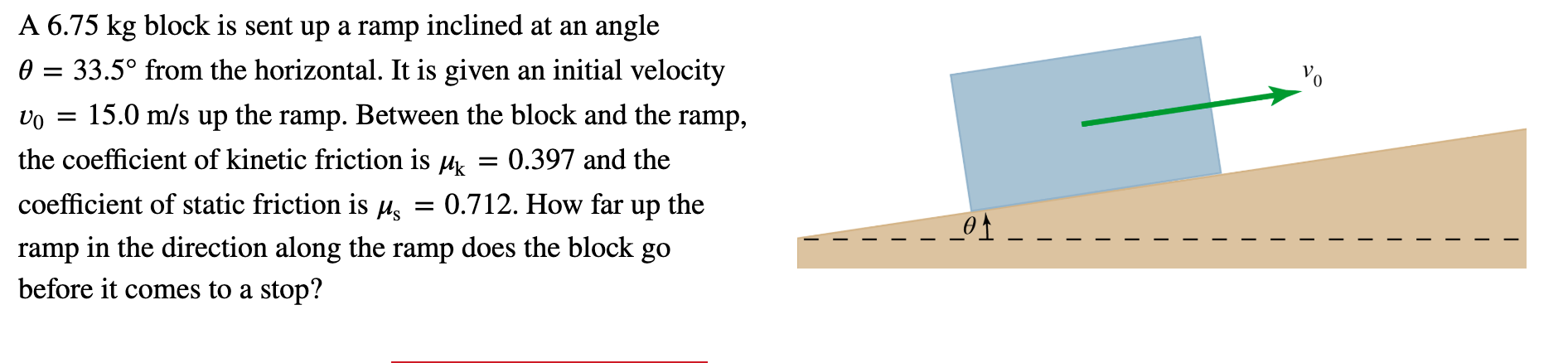A 6.75 kg block is sent up a ramp inclined at an angle 0 = 33.5° from the horizontal. It is given an initial velocity Vo = 15.0 m/s up the ramp. Between the block and the ramp, the coefficient of kinetic friction is uk = 0.397 and the coefficient of static friction is us = 0.712. How far up the ramp in the direction along the ramp does the block go before it comes to a stop? - -...

• ### A 7.55-kg box is pulled up a ramp that is inclined at an angle of 39.0°...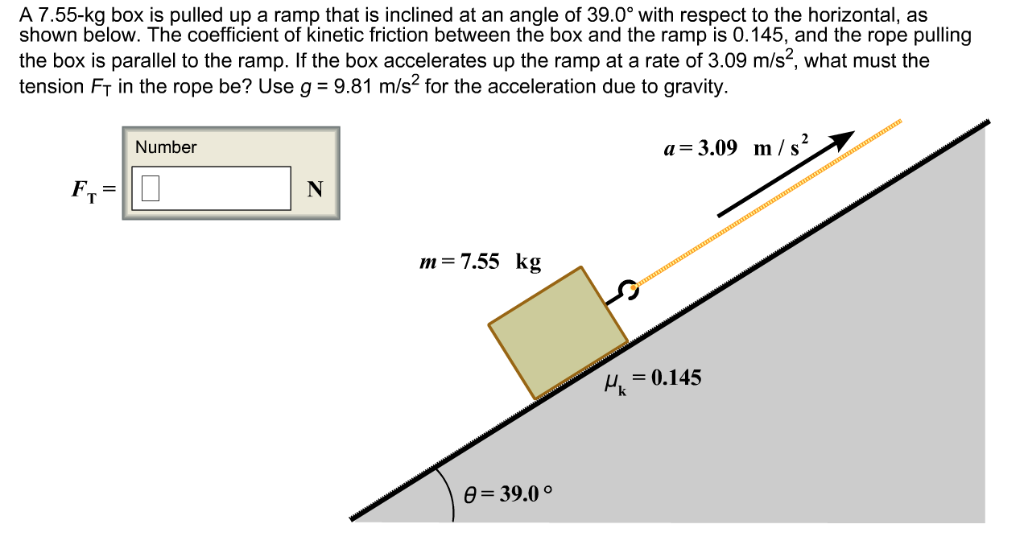A 7.55-kg box is pulled up a ramp that is inclined at an angle of 39.0° with respect to the horizontal, as shown below. The coefficient of kinetic friction between the box and the ramp is 0.145, and the rope pulling the box is parallel to the ramp. If the box accelerates up the ramp at a rate of 3.09 m/s2, what must the tension Fr in the rope be? Use g 9.81 m/s2 for the acceleration due to gravity....

• ### oblem 2: A 5-kg box slides down a ramp. The ramp is l m in length...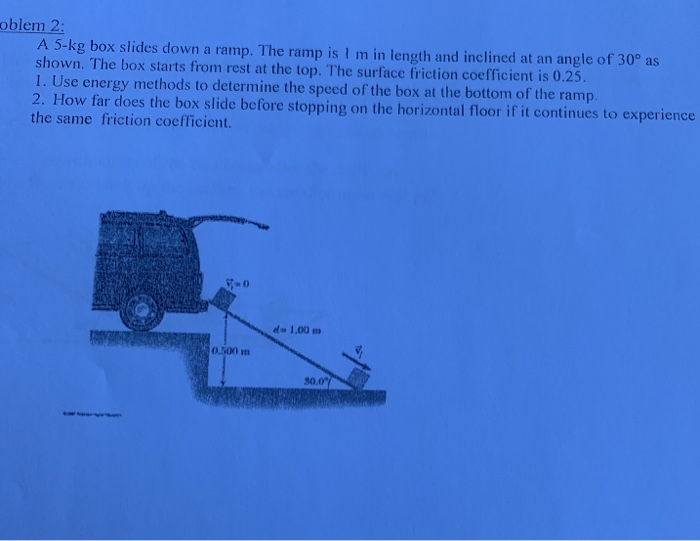oblem 2: A 5-kg box slides down a ramp. The ramp is l m in length and inclined at an angle of 30° as shown. The box starts from rest at the top. The surface friction coefficient is 0.25 1. Use energy methods to determine the speed of the box at the bottom of the ramp 2. How far does the box slide before stopping on the horizontal floor if it continues to experience the same friction coefficient. 1.00 m...

• ### A 3.25-kg block is sent up a ramp inclined at an angle theta = 37.5degree from...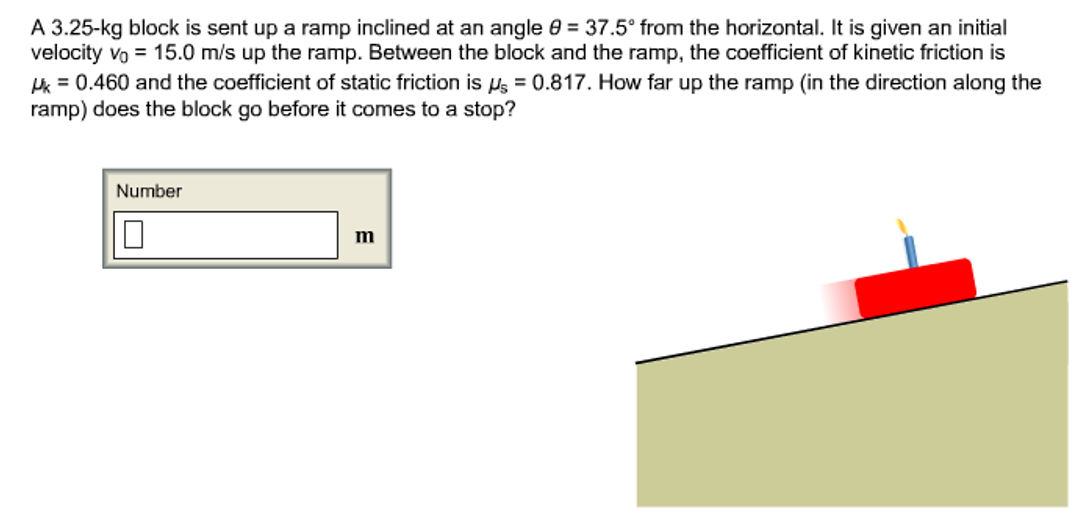A 3.25-kg block is sent up a ramp inclined at an angle theta = 37.5degree from the horizontal. It is given an initial velocity v_0 = 15.0 m/s up the ramp. Between the block and the ramp, the coefficient of kinetic friction is mu _k = 0.460 and the coefficient of static friction is mu _s = 0.817. How far up the ramp (in the direction along the ramp) does the block go before it comes to a stop?

• ### 05-kg box is pulled up a ramp that is inclined at an angle of 33.0° with...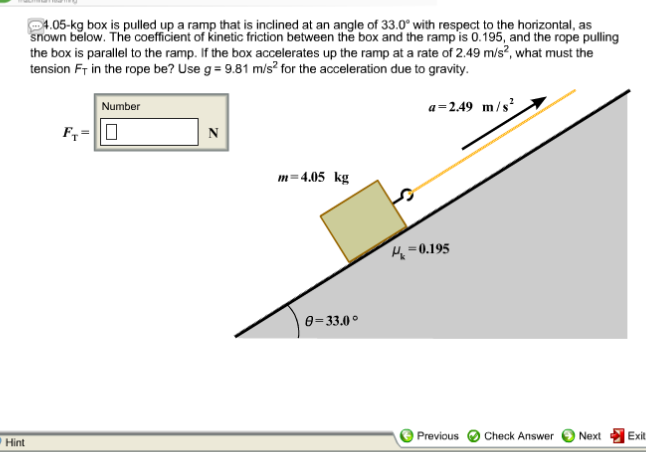05-kg box is pulled up a ramp that is inclined at an angle of 33.0° with respect to the horizontal, as nown below. The coefficient of kinetic friction between the box and the ramp is 0.195, and the rope pulling the box is parallel to the ramp. If the box accelerates up the ramp at a rate of 2.49 m/s2, what must the tension Fr in the rope be? Use g- 9.81 m/s2 for the acceleration due to gravity a-2.49...

• ### A block with mass 12.0 kg is on a ramp that is inclined at an angle...

A block with mass 12.0 kg is on a ramp that is inclined at an angle of 50.0 degrees above the horizontal. The block is accelerating up the ramp at 3.0 m/s2 as you pull on it with a string parallel to the surface of the ramp with a constant force of 140 N.\ A) Draw a free body diagram for the block moving up the ramp and choose a convenient xy-coordinate system to apply Newton's laws of motion. B)...

• ### A 107 kN crate slides down a ramp inclined at 11.1 degrees above horizontal. The initial...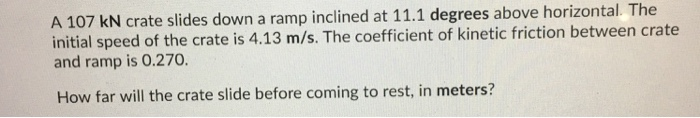A 107 kN crate slides down a ramp inclined at 11.1 degrees above horizontal. The initial speed of the crate is 4.13 m/s. The coefficient of kinetic friction between crate and ramp is 0.270. How far will the crate slide before coming to rest, in meters?

• ### Problem#5: A box with mass 12,0 kg moves on a ramp that is inclined at an...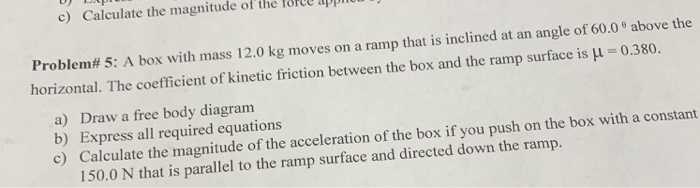Problem#5: A box with mass 12,0 kg moves on a ramp that is inclined at an angle of 60.0 above the horizontal. The coefficient of kinetic friction between the box and the ramp surface is M = 0.380. a) Draw a free body diagram b) Express all required equations c) Calculate the magnitude of the acceleration of the box if you push on the box with a constant 150.0 N that is parallel to the ramp surface and directed down...

• ### A 8.25kg block is sent up a ramp inclined at an angle 38.5 from the horizontal....

A 8.25kg block is sent up a ramp inclined at an angle 38.5 from the horizontal. It is given an initial velocity v0=15.0 m/s up the ramp. Between the block and the ramp, the coefficient of kinetic friction is ?k=0.477 and the coefficient of static friction is ?s= 0.845. HOw far up the ramp (in the direction along the ramp) does the block go before it comes to a stop? _______m